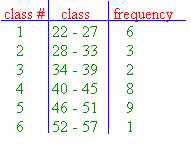### CFA Practice Question

There are 985 practice questions for this topic.

### CFA Practice Question

For the grouped frequency distribution shown below, which of the following is false?A. The median is in class #3.
B. The median is in class #4.
C. The exact value of the minimum cannot be determined.

The only false statement is that the median is in class #3. The position of the median is (n + 1)/2 = (29 + 1)/2 = 15. The 15th piece of data is in class #4. Although we cannot identify the value of the median, we do know that the median is in class #4.

User Comment
haosheng Is the last choice correct?
Nuta It is correct. You do not know the exact value of the minimum. Whether it 22.5 or 22.454688643. :) Therefore it should NOT be chosen as the answer.
Ioannis Exactly. For all you know the minimum could be 27, repeated 6 times to give the class frequency.